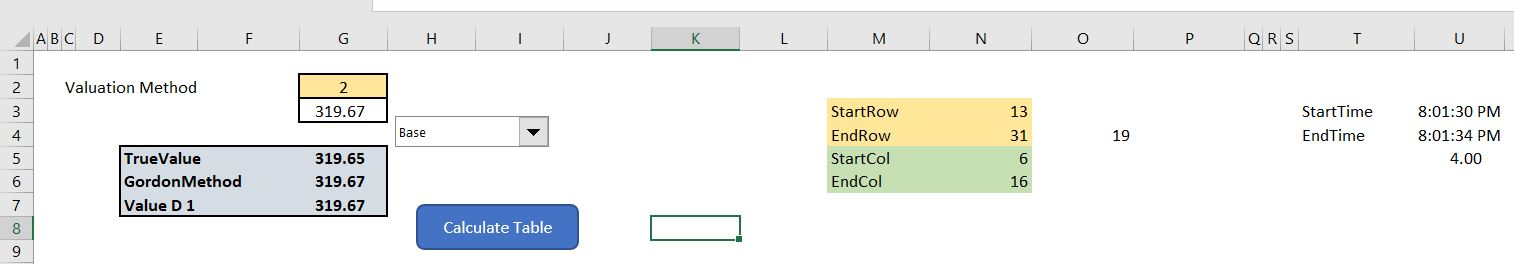# Heavy Financial Models

This page reviews what makes a model heavy — slow to compute; large in terms of size; and difficult to modify in terms of adding new lines and re-structuring pages.  I try to document different strategies for making your file more manageable with time tests and size tests. When re-structuring models I receive from other people, I often find surprising and easy ways to make models operate more quickly through eliminating columns that are mistakenly added to the end of a sheet.  Other things to try are splitting up really long sheets and closing a model after working on it for a while.  On this page I demonstrate how you can test whether things really do make your models less heavy (a term that I really dislike) and I have created a large file that operates slowly to test things.  I also demonstrate that things like using an entire row in the lookup function does not increase the size of a file or reduce the speed of operation.

## Testing the Speed of a Model with a Macro

You can create a macro with the Time and Size statement.  Then you can put the issue that make your model calculations operate slowly or that make you models difficult to structure (add lines and so forth).  You can also test how large your models are before and after you do something like accidently go to the end of the sheet.   The file and the macros shown below illustrate the test I have tried to make for evaluating whether using an entire line in the LOOKUP function makes the file heavier than if you lock in the input cells and do not use the entire row or column.  I have made the file so that you can try different equations and then I make a loop that goes around and re-calculates many times.  In the exercise below, I have tried the following four scenarios:

1. No Equation
2. The look up equation with fixed values (e.g. LOOKUP(B5,\$A\$7:\$K\$7,\$A\$8:\$K\$8))
3. The look up equation with total rows (e.g. LOOKUP(B5,A:A,K:K))
4. The lookup interpolate UDF function (e.g. INTERPOLATE_LOOKUP(B5,A:A,K:K))

The manner in which the file for testing speed works is illustrated in the screenshot below.  The top part of the screenshot demonstrates the inputs for the test.  The second part of the screenshot demonstrates that outputs for the file size and the time taken for calculation for the scenario.  After the scenario is run with different equations, the tests for the size and calculation time can be copied.

You can also test the speed of alternative ways to run a macro as illustrated in the macro below.  Note how the TIME function is used before and after the macro below.```Sub table()

Range("StartTime") = Time
Dim output() As Double
Dim Rows, Columns As Single
Dim Cost_of_Equity As Single
Dim array_size As Single

Cost_of_Equity = Range("Applied").Cells(9, 1)
Terminal_Value = Range("Applied").Cells(6, 1)
Rows = Range("endrow") - Range("startrow") + 1
Columns = Range("endcol") - Range("startcol") + 1

array_size = Columns * Rows

'MsgBox Rows & " " & Columns
Range(Cells(Range("startrow"), Range("endcol")), Cells(Range("endrow"), Range("endcol"))).Select

'Range(Cells(13, 6), Cells(31, 16)).ClearContents
' Selection.ClearContents

ReDim output(Rows, Columns) As Double

Application.ScreenUpdating = False

RowCounter = 0

For Row = Range("startrow") To Range("endrow")
RowCounter = RowCounter + 1
ColumnCounter = 0

Range("Applied").Cells(9, 1) = Cells(Row, Range("startcol") - 1)

For Column = Range("startcol") To Range("endcol")
ColumnCounter = ColumnCounter + 1

Range("Applied").Cells(6, 1) = Cells(Range("startrow") - 1, Column)

'Cells(Row, Column) = Range("truevalue")
'MsgBox Range("truevalue")

output(RowCounter, ColumnCounter) = Range("truevalue")

Next Column

Next Row

Range("table_output") = output

Range("Applied").Cells(9, 1) = Cost_of_Equity
Range("Applied").Cells(6, 1) = Terminal_Value

Range("EndTime") = Time
End Sub

```

## Removing Columns at the End of a Sheet

I have noticed that when people hide columns at the end of a sheet, there is often some little problem with something getting to the end.  Further, there may be some kind of shape such as a button that somehow is at the end of the sheet.  Excel tells you to delete all of the columns at the end of the sheet and then save the file. When there is a shape somewhere, this does not work and the message shown below appears.